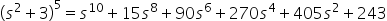Maths-
General
Easy

Question

# Use binomial theorem to expand (s2 + 3)5Hint:

## The correct answer is: 243

### Step 1 of 2:The given expression is (s2 + 3)5  , here x = s2 & y = 3 . The value of n=5, hence there are 5+1=6 terms in the expressions.Step 2 of 2:Substitute the values of (s2 + 3)5 in the binomial equation to get the expansion:Thus, the expansion is:For the expansion of an expression (x + y)n , we would have n+1 terms. This is something you need to keep in mind.#### With Turito Foundation.#### Get an Expert Advice From Turito.